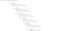# Make your life easier with JavaScript promises

`function(e, res){   if (e) { console.log(e) }}`The full function — and all its nested callbacks — is way too big for me to show here.

## Why is this better than using callbacks?

`flow.procedureC().then(flow.procedureB).then((res) => (   flow.procedureD(res)));`

# Javascript context

`flow.procedureC().then(flow.procedureB)`

## Binding

`flow.procedureC().then(flow.procedureB.bind(flow));`

## Using closures:

`flow.procedureC().then(() => {    return flow.procedureB()});`

# Sharing results

`let res_a, res_b, res_c;a().then((res) => {   res_a = res}).then((res) => {   return b();}).then((res) => {   res_b = res;}).then(c).then(() => {   res_a.callSomething()})`
`.then((res) => {      res_b = res // Saving for later re-use})`

Written by